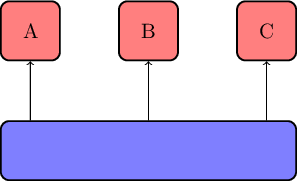# TikZ node positioning

Drawing nodes in TikZ above each other can be tricky. For example, if you want to draw several nodes above another node in an aligned way.

One way to achieve this is as follows.

```\node[minimum width=5cm] (basis) {};
\node[above=of basis.north west, anchor=south west] (a) {A};
\node[above=of basis] (b) {B};
\node[above=of basis.north east, anchor=south east] (c) {C};
```In this way, the left border of A and the right border of B do not extend beyond the basis node. Note that you need to have the `positioning` TikZ libary loaded.

To draw the edges in a vertical fashion, use the intersection shorthand for the nonstandard nodes:

```\draw[->] (basis.north -| a) -- (a);
\draw[->] (basis) -- (b);
\draw[->] (basis.north -| c) -- (c);
```

### no responses for TikZ node positioning

This site uses Akismet to reduce spam. Learn how your comment data is processed.